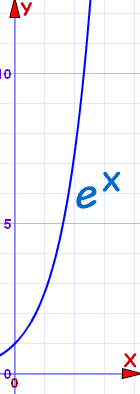Definition of

# Exponential GrowthWhere a value increases in proportion to its current value. Such as always doubling.

The general formula is:

y(x) = abkx

Notice the variable "x" on the right hand side is part of the exponent (hence "exponential").

Example: y = ex is a simple exponential function.